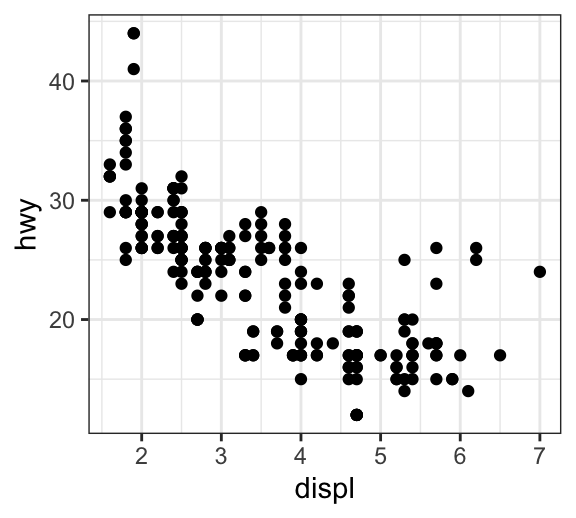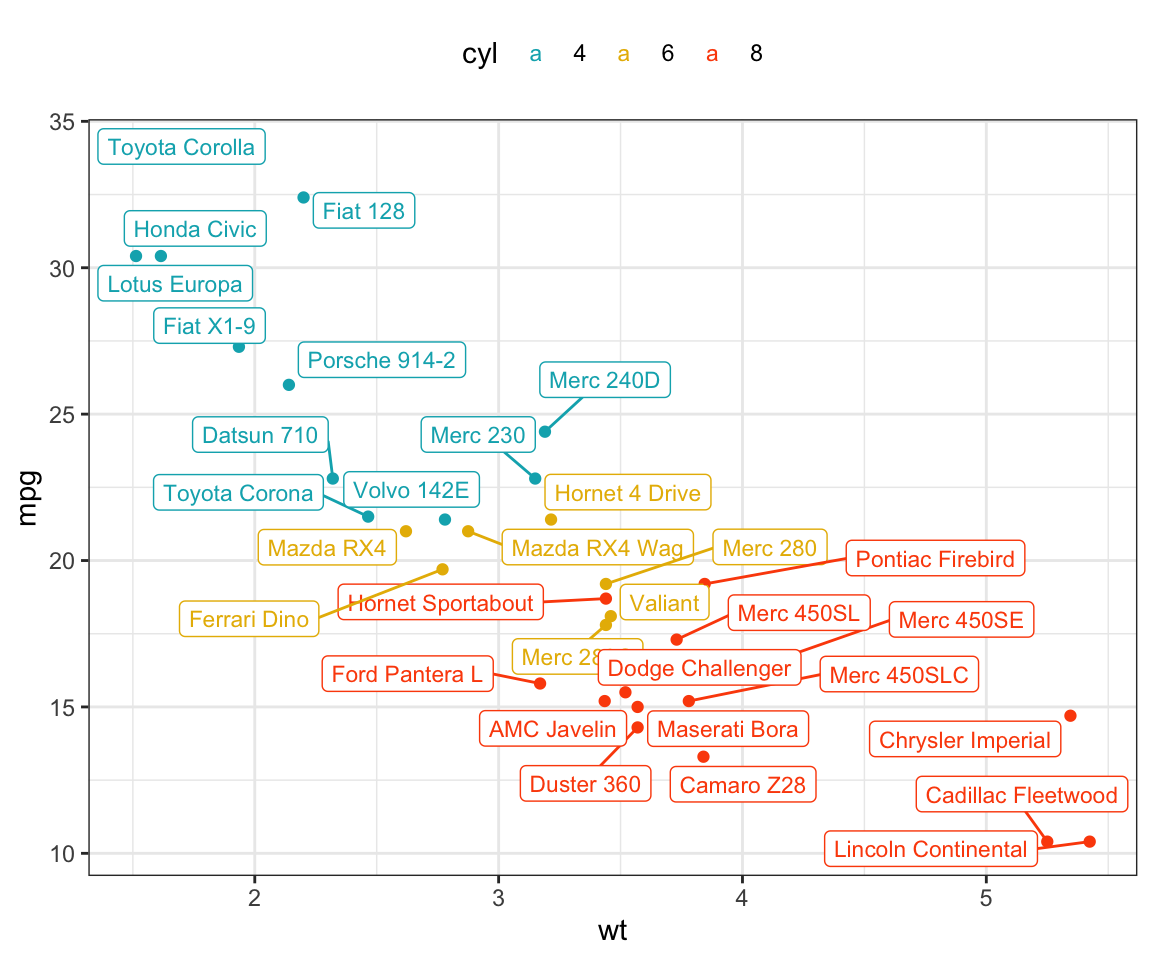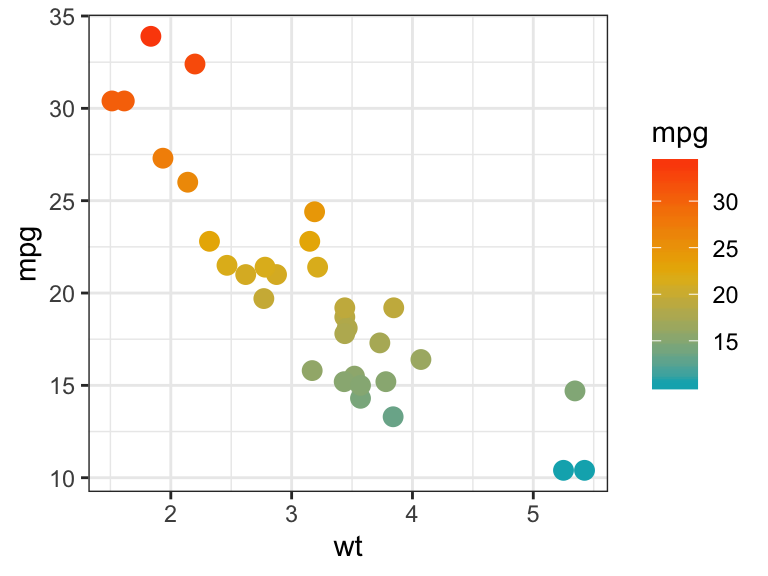# Data Visualization using GGPlot2

## GGPlot Scatter Plot

A Scatter plot (also known as X-Y plot or Point graph) is used to display the relationship between two continuous variables x and y.

By displaying a variable in each axis, it is possible to determine if an association or a correlation exists between the two variables.

The correlation can be: positive (values increase together), negative (one value decreases as the other increases), null (no correlation), linear, exponential and U-shaped.

This article describes how to create scatter plots in R using the ggplot2 package.

You will learn how to:

• Color points by groups
• Create bubble charts
• Add regression line to a scatter plot

Contents:

#### Related Book

GGPlot2 Essentials for Great Data Visualization in R

## Data preparation

Demo dataset: mtcars. The variable cyl is used as grouping variable.

# Load data
data("mtcars")
df <- mtcars

# Convert cyl as a grouping variable
df$cyl <- as.factor(df$cyl)

# Inspect the data
head(df[, c("wt", "mpg", "cyl", "qsec")], 4)
##                  wt  mpg cyl qsec
## Mazda RX4      2.62 21.0   6 16.5
## Mazda RX4 Wag  2.88 21.0   6 17.0
## Datsun 710     2.32 22.8   4 18.6
## Hornet 4 Drive 3.21 21.4   6 19.4

Load the ggplot2 package and set the default theme to theme_bw() with the legend at the top of the plot:

library(ggplot2)
theme_set(
theme_bw() +
theme(legend.position = "top")
)

## Basic scatter plots

• Key functions: geom_point() for creating scatter plots.
• Key arguments: color, size and shape to change point color, size and shape.
# Initiate a ggplot
b <- ggplot(df, aes(x = wt, y = mpg))

# Basic scatter plot
b + geom_point()

# Change color, shape and size
b + geom_point(color = "#00AFBB", size = 2, shape = 23)The different point shapes commonly used in R, include:## Scatter plots with multiple groups

This section describes how to change point colors and shapes by groups. The functions scale_color_manual() and scale_shape_manual() are used to manually customize the color and the shape of points, respectively.

In the R code below, point shapes and colors are controlled by the levels of the grouping variable cyl :

# Change point shapes by the levels of cyl
b + geom_point(aes(shape = cyl))

# Change point shapes and colors by the levels of cyl
# Set custom colors
b + geom_point(aes(shape = cyl, color = cyl)) +
scale_color_manual(values = c("#00AFBB", "#E7B800", "#FC4E07"))• Key R function: geom_smooth() for adding smoothed conditional means / regression line.
• Key arguments:
• color, size and linetype: Change the line color, size and type.
• fill: Change the fill color of the confidence region.

A simplified format of the function geom_smooth():

geom_smooth(method="auto", se=TRUE, fullrange=FALSE, level=0.95)
• method : smoothing method to be used. Possible values are lm, glm, gam, loess, rlm.
• method = “loess”: This is the default value for small number of observations. It computes a smooth local regression. You can read more about loess using the R code ?loess.
• method =“lm”: It fits a linear model. Note that, it’s also possible to indicate the formula as formula = y ~ poly(x, 3) to specify a degree 3 polynomial.
• se : logical value. If TRUE, confidence interval is displayed around smooth.
• fullrange : logical value. If TRUE, the fit spans the full range of the plot
• level : level of confidence interval to use. Default value is 0.95

To add a regression line on a scatter plot, the function geom_smooth() is used in combination with the argument method = lm. lm stands for linear model.

# Add regression line
b + geom_point() + geom_smooth(method = lm)

# Point + regression line
# Remove the confidence interval
b + geom_point() +
geom_smooth(method = lm, se = FALSE)

# loess method: local regression fitting
b + geom_point() + geom_smooth()Change point color and shapes by groups:

# Change color and shape by groups (cyl)
b + geom_point(aes(color = cyl, shape=cyl)) +
geom_smooth(aes(color = cyl, fill = cyl), method = lm) +
scale_color_manual(values = c("#00AFBB", "#E7B800", "#FC4E07"))+
scale_fill_manual(values = c("#00AFBB", "#E7B800", "#FC4E07"))

# Remove confidence intervals
# Extend the regression lines: fullrange
b + geom_point(aes(color = cyl, shape = cyl)) +
geom_smooth(aes(color = cyl), method = lm, se = FALSE, fullrange = TRUE) +
scale_color_manual(values = c("#00AFBB", "#E7B800", "#FC4E07"))+
scale_fill_manual(values = c("#00AFBB", "#E7B800", "#FC4E07"))## Add marginal rugs to a scatter plot

The function geom_rug() is used to display display individual cases on the plot.

# Add marginal rugs
b + geom_point() + geom_rug()

# Change colors by groups
b + geom_point(aes(color = cyl)) +
geom_rug(aes(color = cyl))

# Add marginal rugs using faithful data
data(faithful)
ggplot(faithful, aes(x = eruptions, y = waiting)) +
geom_point() + geom_rug()## Jitter points to reduce overplotting

The mpg data set [in ggplot2] is used in the following examples.

To reduce overplotting, the option position = position_jitter() with the arguments width and height are used:

• width: degree of jitter in x direction.
• height: degree of jitter in y direction.
# Default plot
ggplot(mpg, aes(displ, hwy)) +
geom_point()

# Use jitter to reduce overplotting
ggplot(mpg, aes(displ, hwy)) +
geom_point(position = position_jitter(width = 0.5, height = 0.5))Key functions:

• geom_text() and geom_label(): ggplot2 standard functions to add text to a plot.
• geom_text_repel() and geom_label_repel() [in ggrepel package]. Repulsive textual annotations. Avoid text overlapping.

First install ggrepel (ìnstall.packages("ggrepel")), then type this:

library(ggrepel)

# Add text to the plot
.labs <- rownames(df)
b + geom_point(aes(color = cyl)) +
geom_text_repel(aes(label = .labs,  color = cyl), size = 3)+
scale_color_manual(values = c("#00AFBB", "#E7B800", "#FC4E07"))# Draw a rectangle underneath the text, making it easier to read.
b + geom_point(aes(color = cyl)) +
geom_label_repel(aes(label = .labs,  color = cyl), size = 3)+
scale_color_manual(values = c("#00AFBB", "#E7B800", "#FC4E07"))## Bubble chart

In a bubble chart, points size is controlled by a continuous variable, here qsec. In the R code below, the argument alpha is used to control color transparency. alpha should be between 0 and 1.

b + geom_point(aes(color = cyl, size = qsec), alpha = 0.5) +
scale_color_manual(values = c("#00AFBB", "#E7B800", "#FC4E07")) +
scale_size(range = c(0.5, 12))  # Adjust the range of points size## Color by a continuous variable

• Color points according to the values of the continuous variable: “mpg”.
• Change the default blue gradient color using the function scale_color_gradientn() [in ggplot2], by specifying two or more colors.
b + geom_point(aes(color = mpg), size = 3) +
scale_color_gradientn(colors = c("#00AFBB", "#E7B800", "#FC4E07")) +
theme(legend.position = "right")`